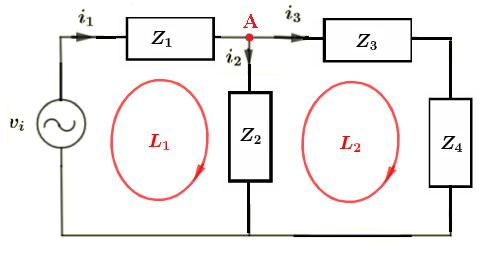# AC Kirchhoff's Equations Solver and Calculator

  
An online calculator to solve Kirchhoff's equations in ac circuits, using complex numbers, is presented. The calculator accepts any number of equations. The outputs are the values of all currents in the given circuit.

## Write the voltages and Currents Equations Using Kirchhoff's and Solve

Example
An example of 3 equations is done for you and you may follow it at the start before using the calculator for other circuits that may involve any number of equations.
Find the currents through the impedances in the circuit below, given the voltage sources $v_i = 9 \angle 0$ Volts, $Z_1 = 15 + j 10 \; \Omega$ , $Z_2 = 4.8 - j 3.6 \; \Omega$, $Z_3 = - j5 \; \Omega$ and $Z_4 = 7.5 + j 7.5 \; \Omega$.step 1 - Use Kirchhoff's laws of voltages to write an equation for each closed loop:
Loop L1:     $v_i - Z_1 i_1 - Z_2 i_2 = 0$
Loop L2:     $Z_2 i_2 - Z_3 i_3 - Z_4 i_3 = 0$
step 2: Use Kirchhoff's laws of currents to write an equation at each node:
Node A:     $i_1 - i_2 - i_3 = 0$
step 2 - Rearrange the equations so that terms depending on the unknowns $i_1, i_2$ and $i_3$ are on the left and all constants on the right, and set the unknowns in the same order in all equations:
$\begin{array}{lclcl} Z_1 i_1 + Z_2 i_2 & = & v_i \\ Z_2 i_2 - (Z_3 + Z_4) i_3 & = & 0 \\ i_1 - i_2 - i_3 & = & 0 \end{array}$
step 3 - Substitute all impedances and voltages of voltage sources by their numerical values and write all unknowns in the equations including the ones whose coefficents are zeros:
$\begin{array}{lclcl} (15 + j 10)\; i_1 + (4.8 - j 3.6) \; i_2 + 0 \; i_3& = & 9 \\ 0 \; i_1 + (4.8 - j 3.6) i_2 - (7.5 + j 2.5) i_3 & = & 0 \\ i_1 - i_2 - i_3 & = & 0 \end{array}$
step 4 - Enter the number of equations $m$ ( which is equal to the number of unknowns) and the coefficients of $i_1, i_2$ and $i_3$ as complex numbers of the form "(real part , imaginary part)" into the calculator and calculate the currents which are given in complex and polar forms:
Enter Number of Equations: $m =$     Number of Decimals:

Change values of coefficients in above matrix (if needed) and click